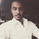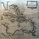Dilihat 2886
Double Bollinger Strategy. Price crosses inner BB (with increasing outer BB) long is placed. Long is closed when price closes below outer BB. Reversed for short. The numbers are tuned for NZDUSD 15min chart.

Pbin = percent on inner BB
Pbout = percent on outer BB

There are all kinds of other inputs, trailing stop, limit, etc.

Errors, questions, suggestions are wanted, thank you
```//@version=2
strategy("BB Strat",default_qty_type = strategy.percent_of_equity, default_qty_value = 100,currency="USD",initial_capital=100, overlay=true)
l=input(title="length",defval=100)
pbin=input(type=float,step=.1,defval=.25)
pbout=input(type=float,step=.1,defval=1.5)
ma=sma(close,l)
sin=stdev(ma,l)*pbin
sout=stdev(ma,l)*pbout
inu=sin+ma
inb=-sin+ma
outu=sout+ma
outb=-sout+ma
plot(inu,color=lime)
plot(inb,color=lime)
plot(outu,color=red)
plot(outb,color=yellow)

inpTakeProfit = input(defval = 0, title = "Take Profit", minval = 0)
inpStopLoss = input(defval = 0, title = "Stop Loss", minval = 0)
inpTrailStop = input(defval = 0, title = "Trailing Stop Loss", minval = 0)
inpTrailOffset = input(defval = 0, title = "Trailing Stop Loss Offset", minval = 0)
useTakeProfit = inpTakeProfit >= 1 ? inpTakeProfit : na
useStopLoss = inpStopLoss >= 1 ? inpStopLoss : na
useTrailStop = inpTrailStop >= 1 ? inpTrailStop : na
useTrailOffset = inpTrailOffset >= 1 ? inpTrailOffset : na

longCondition = close>inu and rising(outu,1)
exitlong = (open>outu and close<outu) or crossunder(close,ma)

shortCondition = close<inb and falling(outb,1)
exitshort = (open<outb and close>outb) or crossover(close,ma)

strategy.entry(id = "Long", long=true, when = longCondition)
strategy.close(id = "Long", when = exitlong)
strategy.exit("Exit Long", from_entry = "Long", profit = useTakeProfit, loss = useStopLoss, trail_points = useTrailStop, trail_offset = useTrailOffset, when=exitlong)

strategy.entry(id = "Short", long=false, when = shortCondition)
strategy.close(id = "Short", when = exitshort)
strategy.exit("Exit Short", from_entry = "Short", profit = useTakeProfit, loss = useStopLoss, trail_points = useTrailStop, trail_offset = useTrailOffset, when=exitshort)```

## Komentarhi. how can you make it to t/p at +30 pips and s/l at -15 ??
JawabYoussefRazak
When you add the script to your chart look for a gear symbol near its label on the chart upper left side. When you press the gear symbol you it choices, look for a list that includes stop loss, trailing stop, limit (profit). That is where you enter that information.
Jawabsk12722
The +- is the amount long or short. I dont remember if I was using USD or contracts when I published this script.
Jawab